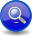Boost C++ Libraries

...one of the most highly regarded and expertly designed C++ library projects in the world.

Sequences (a << b)
Description

Generator sequences are used to consecutively combine different, more primitive generators. All generators in a sequence are invoked from left to right as long as they succeed.

// forwards to <boost/spirit/home/karma/operator/sequence.hpp>
#include <boost/spirit/include/karma_sequence.hpp>

Also, see Include Structure.

Expression Semantics

Semantics of an expression is defined only where it differs from, or is not defined in NaryGenerator.

Expression

Semantics

a << b

The generators a and b are executed sequentially from left to right and as long as they succeed. A failed generator stops the execution of the entire sequence and makes the sequence fail as well.

It is important to note, that sequences don't perform any buffering of the output generated by its elements. That means that any failing sequence might have already generated some output, which is not rolled back.

TipThe simplest way to force a sequence to behave as if it did buffering is to wrap it into a buffering directive (see buffer): buffer[a << b << c] which will not generate any output in case of a failing sequence.
Attributes

Expression

Attribute

a << b (sequence)

a: A, b: B --> (a << b): tuple<A, B>
a: A, b: Unused --> (a << b): A
a: Unused, b: B --> (a << b): B
a: Unused, b: Unused --> (a << b): Unused

a: A, b: A --> (a << b): vector<A>
a: vector<A>, b: A --> (a << b): vector<A>
a: A, b: vector<A> --> (a << b): vector<A>
a: vector<A>, b: vector<A> --> (a << b): vector<A>

ImportantThe table above uses tuple and vector as placeholders only. The notation tuple stands for any fusion sequence of two elements, where A is the type of its first element and B is the type of its second element. The notation of vector stands for any STL container holding elements of type A.

The attribute composition and propagation rules as shown in the table above make sequences somewhat special as they can operate in two modes if all elements have the same attribute type: consuming fusion sequences and consuming STL containers. The selected mode depends on the type of the attribute supplied.

Complexity

The overall complexity of the sequence generator is defined by the sum of the complexities of its elements. The complexity of the sequence itself is O(N), where N is the number of elements in the sequence.

Example
NoteThe test harness for the example(s) below is presented in the Basics Examples section.

Some includes:

#include <boost/spirit/include/karma.hpp>
#include <boost/spirit/include/phoenix_core.hpp>
#include <boost/spirit/include/phoenix_operator.hpp>
#include <boost/fusion/include/std_pair.hpp>
#include <iostream>
#include <string>

Some using declarations:

using boost::spirit::karma::double_;

Basic usage of a sequence:

test_generator_attr("1.0,2.0", double_ << ',' << double_, std::make_pair(1.0, 2.0));